All You Need to Know About

# Lookup Formulas in Excel

Lookup Formulas in Excel are a very core part of your Excel toolkit. Learn about how to perform lookup in Excel here so that you know what to use for each problem!

Here are the top things on what you can do with Lookup in Excel:

## VLOOKUP Example: Vlookup with a Drop Down List

What does it do?

Searches for a value in the first column of a table array and returns a value in the same row from another column (to the right) in the table array.

Formula breakdown:

=VLOOKUP(lookup_value, table_array, col_index_num, [range_lookup])

What it means:

=VLOOKUP(this value, in this list, and get me value in this column, Exact Match/FALSE/0])

The VLOOKUP function in Excel can become interactive and more powerful when applying a Data Validation (drop down menu/list) as the Lookup_Value.  So as you change your selection from the drop down list, the VLOOKUP value also changes.

See how easy it is to apply this with a quick VLOOKUP example below…

STEP 1: Go to Data > Data Validation.STEP 2: Select List in the Allow dropdown

For the Source, ensure that it has the 4 Stock List values selected. Click OK.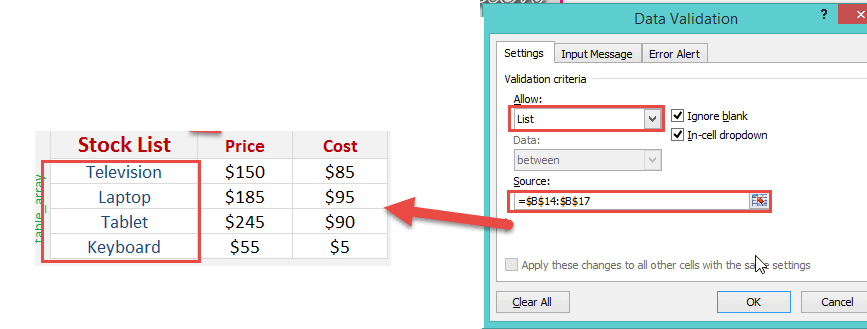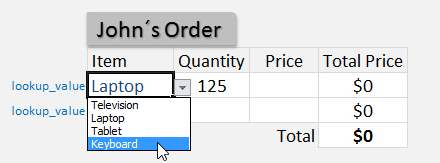STEP 3: We need to enter the Vlookup function:

## +VLOOKUP(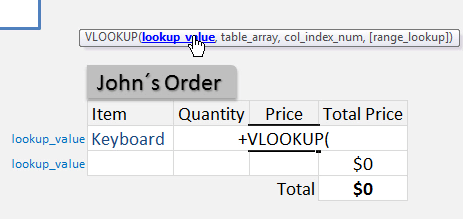The Vlookup arguments:

## lookup_value

What are we looking for?

Reference the cell that contains the text or value:

## =VLOOKUP(G15,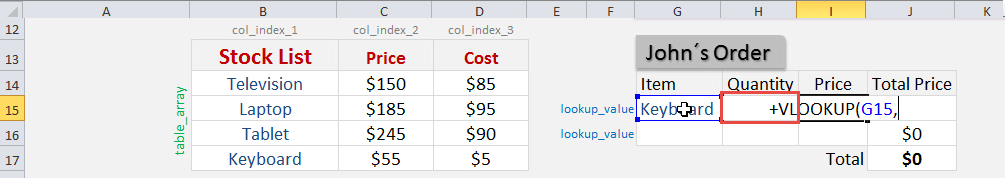## table_array

From which list are we doing a lookup on?

Place in the cell range of the Stock List:

## +VLOOKUP(G15, \$B\$14:\$D\$17,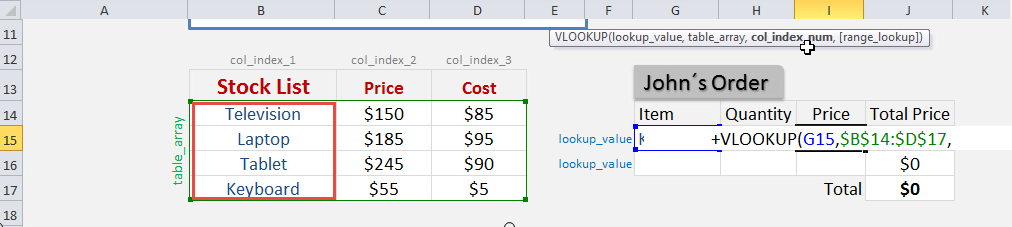## col_index_num

From which column do we want to retrieve the value?

We want to retrieve the Price which is the SECOND column from our table array:

## +VLOOKUP(G15, \$B\$14:\$D\$17, 2,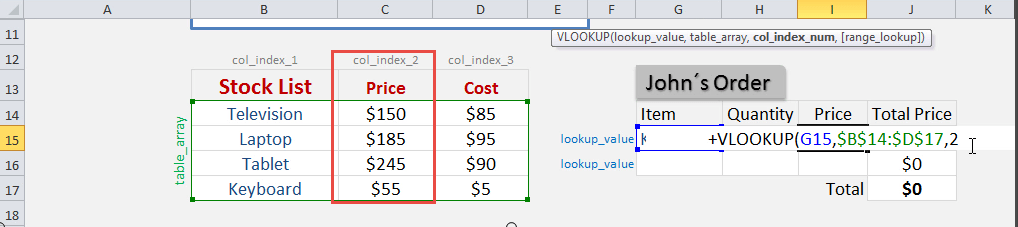## [range_lookup]

Do we want an exact match?

Place in FALSE to signify that we want an exact match:

## +VLOOKUP(G15, \$B\$14:\$D\$17, 2, FALSE)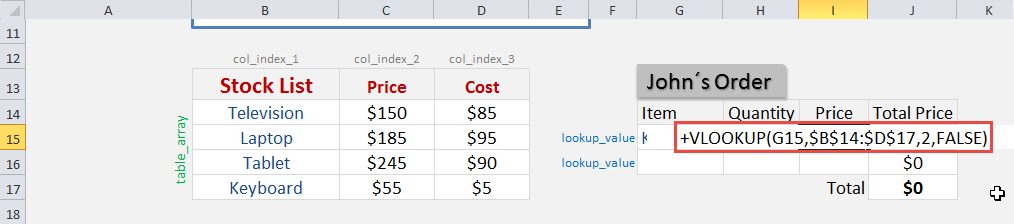The price now dynamically changes based on your selection: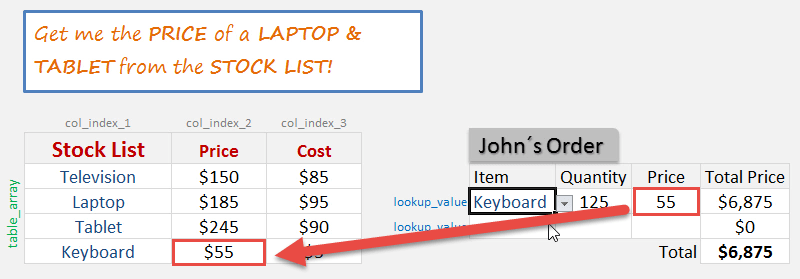## VLOOKUP Multiple Columns

What does it do?

Searches for a value in the first column of a table array and returns the sum of values in the same row from other columns (to the right) in the table array.

Formula breakdown:

{=SUM(VLOOKUP(lookup_value, table_array, {col_index_num1,col_index_num2}, [range_lookup]))}

What it means:

{=SUM(VLOOKUP(this value, in this list, {and sum the value in this column, with the value in this column}, Exact Match/FALSE/0]))}

The VLOOKUP function can be combined with other functions such as the Sum, Max or Average to calculate values in multiple columns.  As this is an array formula, to make it work we simply need to press CTRL+SHIFT+ENTER at the end of the formula.  A very powerful feature for any serious analyst!

We want to get the total number of units for Laptop (16,700 + 18,700 units).

STEP 1: We need to enter the VLOOKUP function in a blank cell:

## =VLOOKUP(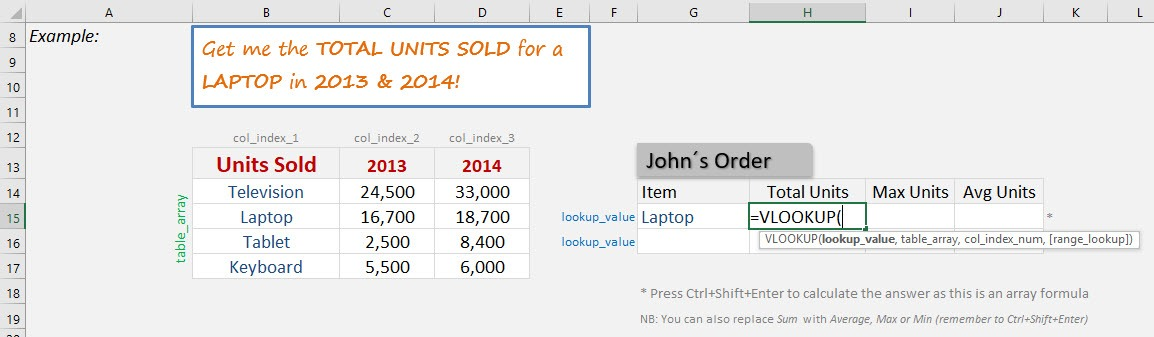STEP 2: The VLOOKUP arguments:

## Lookup_value

What is the value to be looked up?

Select the cell that contains the item name, which is Laptop.

## =VLOOKUP(G15,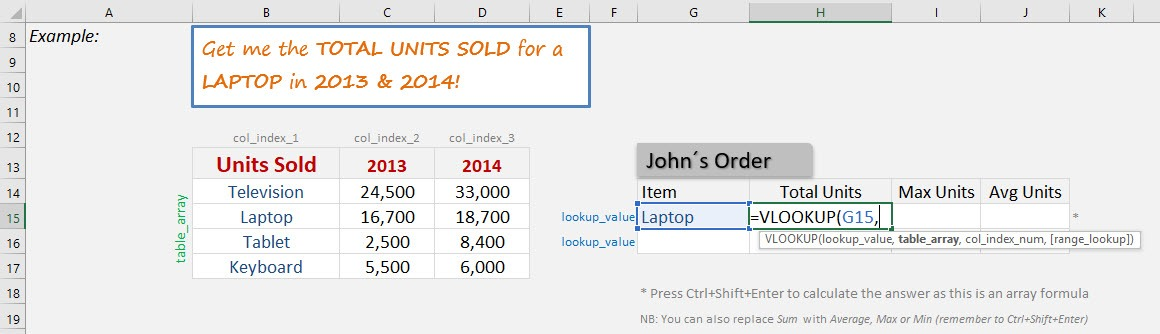## Table_array

Where is the list of data?

Select the Units Sold table, as that is where our formula is going to get the unit numbers.

## =VLOOKUP(G15, B14:D17,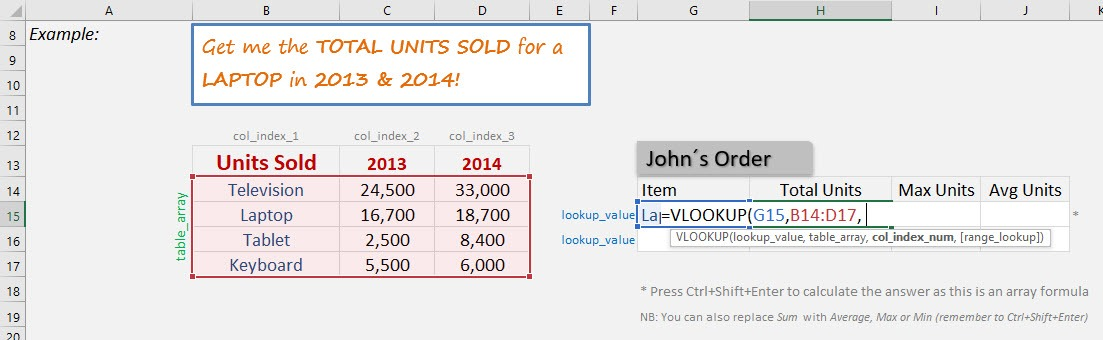## {Col_index_num1, Col_index_num2}

Which columns in the table_array contains the data you want to return?

We want to get the unit numbers of Years 2013 and 2014. So that will be columns 2 and 3.

## =VLOOKUP(G15, B14:D17, {2,3},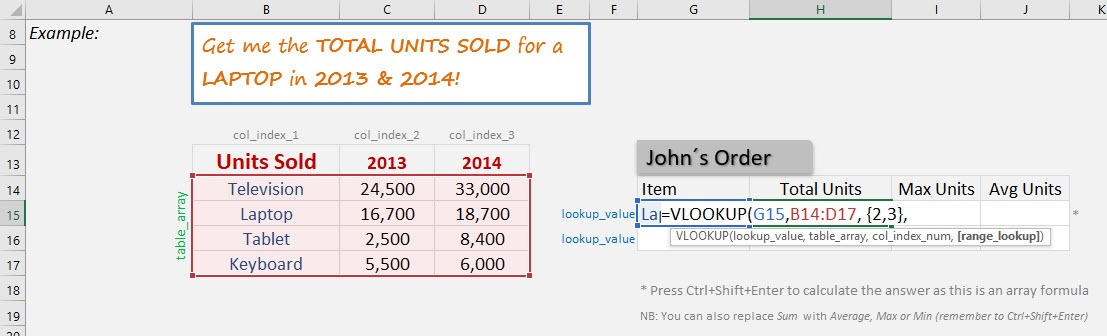## [Range_lookup]

Would it be an approximate match?

Set this to FALSE as we want an exact match for Laptop.

## =VLOOKUP(G15, B14:D17, {2,3}, FALSE)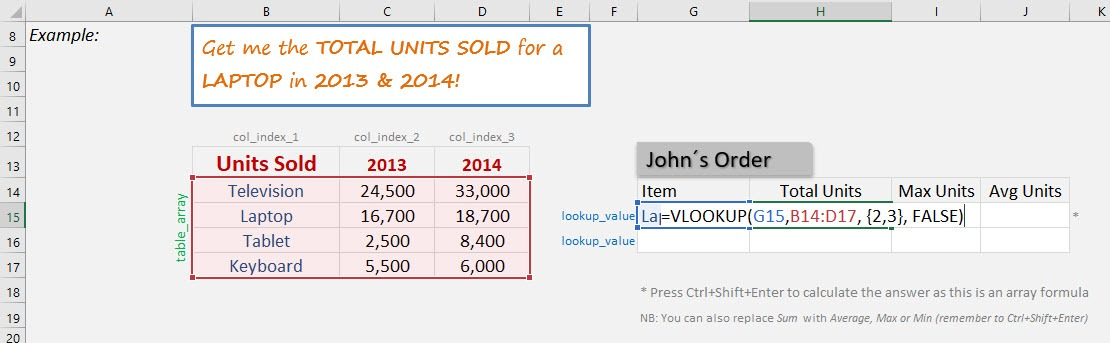STEP 3: Now wrap the formula with the SUM formula as we want to get the total number of sold units for Laptop.

## =SUM(VLOOKUP(G15, B14:D17, {2,3}, FALSE))

Ensure you are pressing CTRL+SHIFT+ENTER as we want to calculate this as an array formula.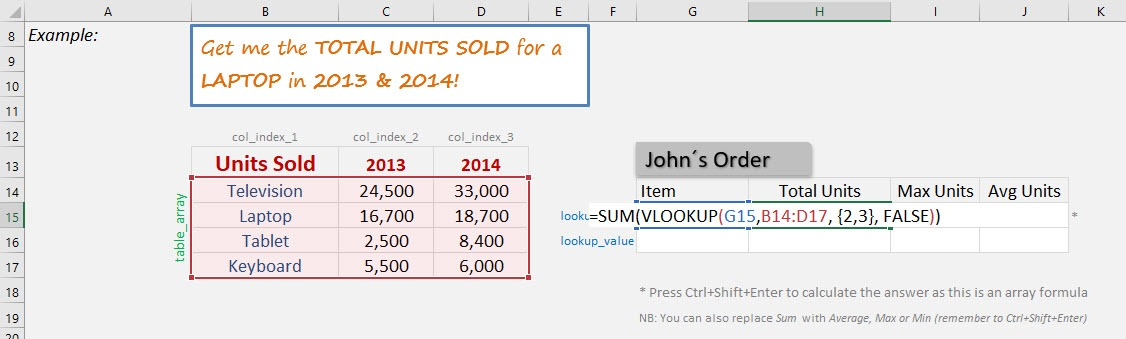Do the exact same formula for Max Units and Average Units, by changing the SUM Formula with the MAX Formula and Average Formula respectively.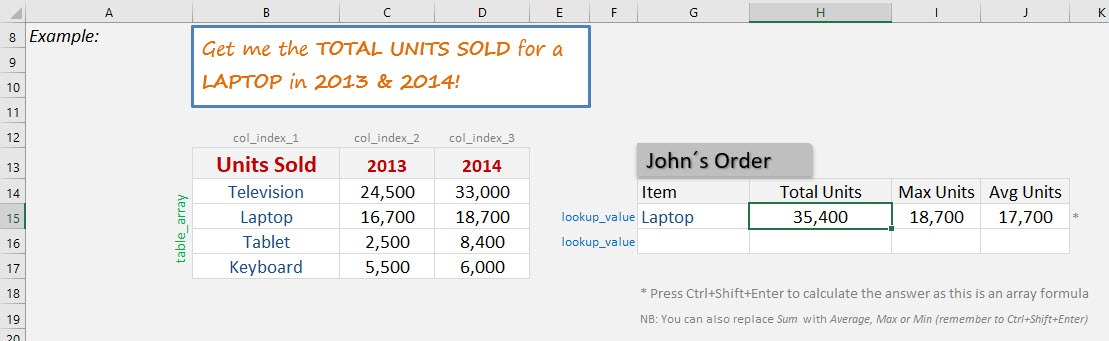## Match Two Lists With The MATCH Function

What does it do?

It returns the position of an item in a range

Formula breakdown:

=MATCH(lookup_valuelookup_array[match_type])

What it means:

=MATCH(lookup this valuefrom this list or range of cellsreturn me the Exact Match).

I am sure that you have come across many occasions where you have two lists of data and want to know if a specific item in List1 exists in List2.

Well I have!

With the MATCH function you can verify if a cell´s item in List1 exists in List2.

The function will return the row position of that item in List2 hence confirming that it exists.  If you get a #N/A it means that the cell´s item does not exist in List2.

You can then go ahead and filter your List1 with either the values returned or the #N/As.

Here are our 2 Lists: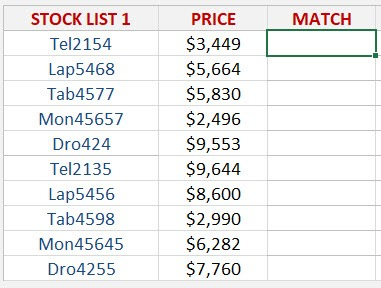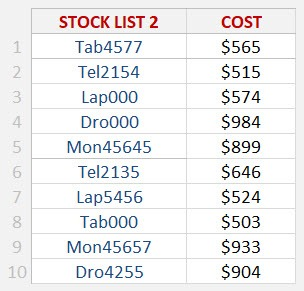STEP 1: We need to enter the MATCH function in a blank cell:

## =MATCH(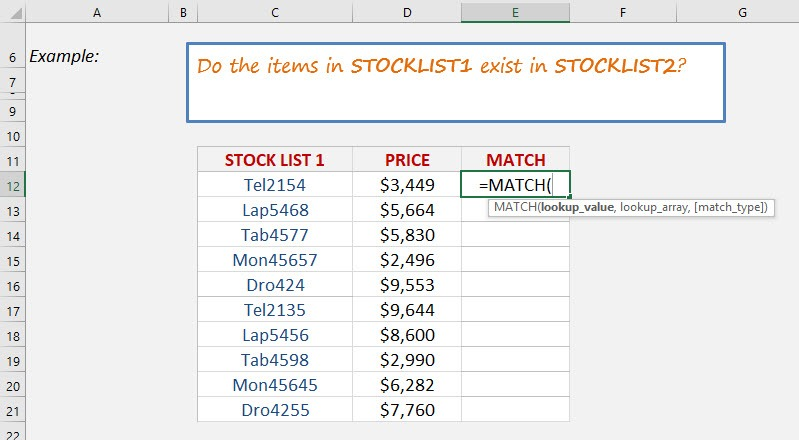STEP 2: The MATCH arguments:

## Lookup_value

What is the value you want to check?

Select the cell containing the List1 value, as this is what we want to check against List2.

## =MATCH(C12,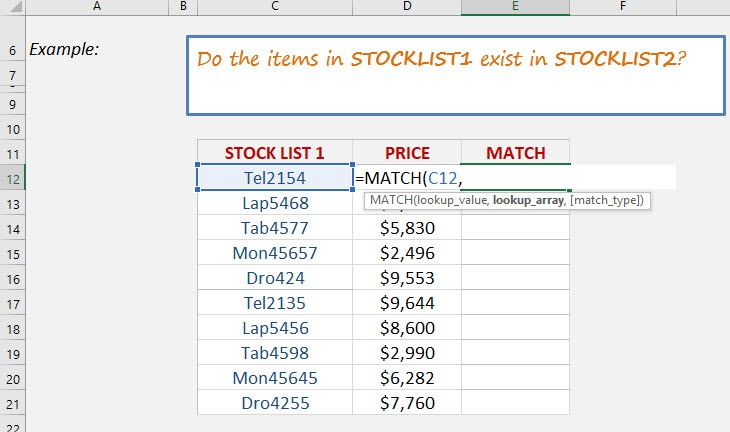## Lookup_array

What is the list you want to check against?

Select the entire List2.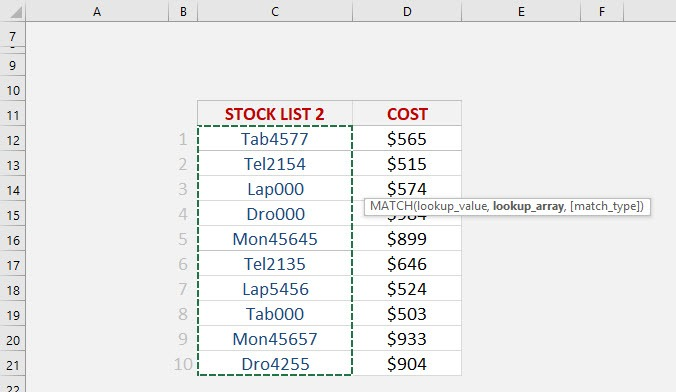And ensure to press F4 to make it an absolute reference.

## =MATCH(C12, list2!\$C\$12:\$C:21,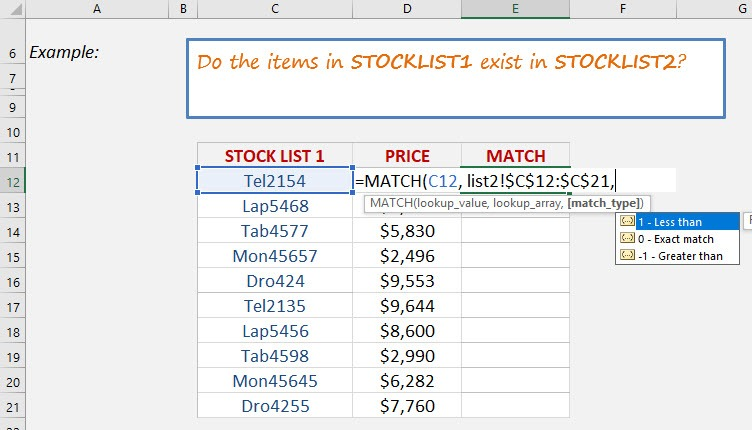## Match_type

We want an exact match so place in 0.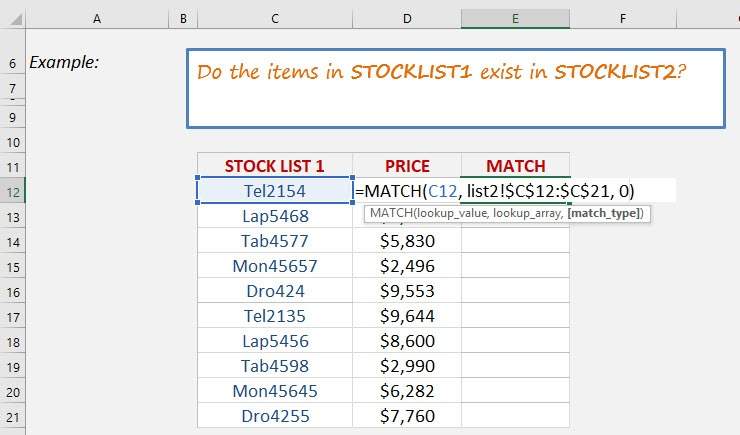Apply the same formula to the rest of the cells by dragging the lower right corner downwards.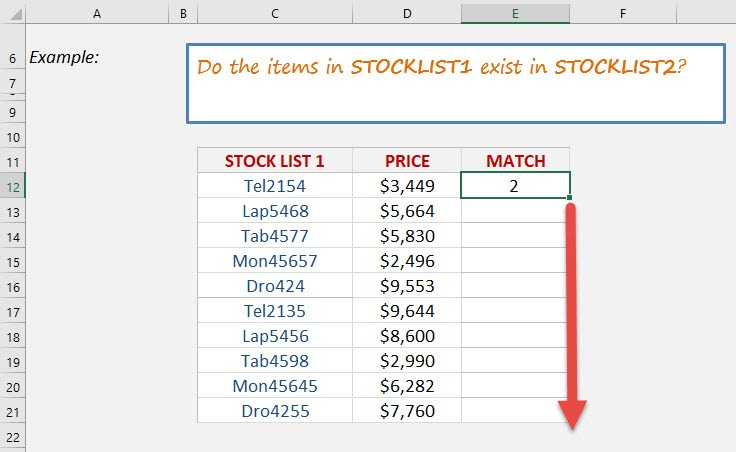You now have all of results! You can see which row numbers the items exist in List2. For example, Mon45657 in List1 exists in List2 Row 9! If it does not exist in List2, then #N/A is displayed.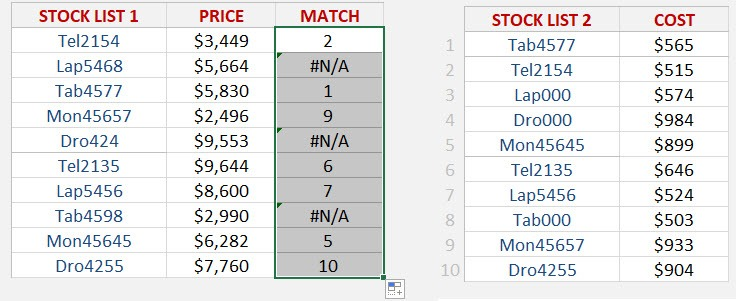Latest Tutorials

June 1, 2021

April 1, 2021

March 31, 2021

March 16, 2021

March 5, 2021

March 3, 2021

March 1, 2021